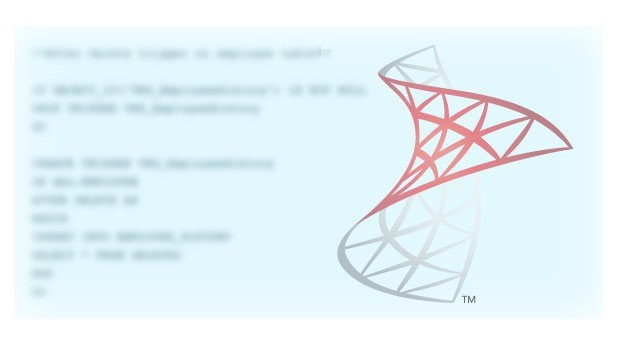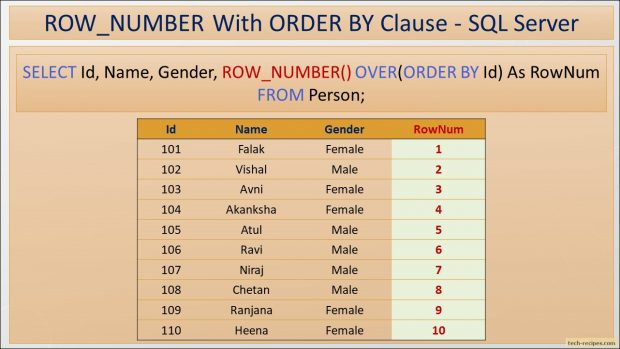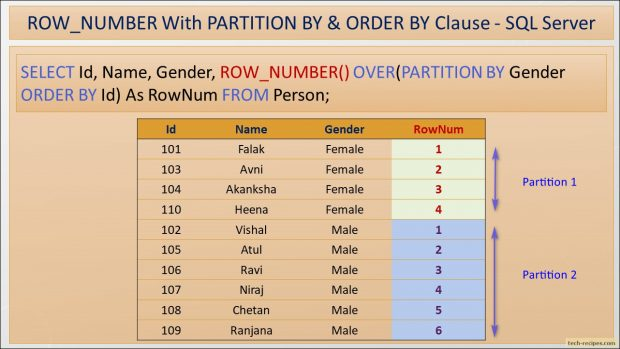# How To Use ROW_NUMBER Function In SQL Server

Posted January 27, 2019 by Vishwanath Dalvi in Database, SQL ServerROW_NUMBER, analytic and ranking function in SQL Server. Assigns sequence number to table rows in incrementing integer values starting at 1 for the first row. Assigned row numbers act as temporary value to result set not persistent. ROW_NUMBER function works with ORDER BY clause to sort the rows in the defined order then numbers the query result set.

For example, we have 10 rows is a table, and using ROW_NUMBER function with ORDER BY clause assigns numbers starting at 1 and ending with 10.Likewise, ROW_NUMBER function also used along with PARTITION BY clause to create a separate window of rows based on conditions. For instance, we have 10 rows in Person table, 5 Males and 6 Females. Using ROW_NUMBER function and PARTITION BY Gender column will assign 1 to 5 numbers to Males and 1 to 6 numbers to Females partition.Syntax

`ROW_NUMBER () OVER(PARTITION BY column_name ORDER BY column_name)`

Arguments

`PARTITION BY - Optional, seperate rows into different partitions and applies ROW_NUMBER function to each partition.ORDER BY - Sorts the row in a defined order and assigns number to query result set.`

### ROW_NUMBER Function – Points to Ponder

1.Assigns incrementing integer values starting at 1.

2.Subsequent rows will get the next higher value, ideally previous row number value + 1.

3.Assigned numbers are temporary not persistent, for persistent number use IDENTITY and SEQUENCE objects.

4.PARTITION BY clause used to create a window of rows which is optional.

5.Multiple columns can be used with ROW_NUMBER and PARTITION BY to create windows and assigning numbers to each window starting at 1.

6.No parameters allowed with ROW_NUMBER.

### ROW_NUMBER Function – Examples

Let’s demonstrate ROW_NUMBER function with helpful and practical examples.

1.

### ROW_NUMBER – With ORDER BY Clause

Following query uses ROW_NUMBER function with ORDER BY Clause on the ID column. RowNum, a derived column name, an alias for the output of ROW_NUMBER function. Sorting the rows by ID column and assigning a number to each row starting with 1 and increasing the value for subsequence rows.

```--ROW_NUMBER - With ORDER BY Clause
USE tempdb;
GO

IF OBJECT_ID('dbo.Person', 'U') IS NOT NULL
DROP TABLE dbo.Person;
GO

CREATE TABLE dbo.Person
(
ID		INT	    PRIMARY KEY
,Name		VARCHAR(50)
,Gender	VARCHAR(6)
);

INSERT INTO dbo.Person
VALUES
(101,'Falak'   ,'Female')
,(102,'Vishal'  ,'Male')
,(103,'Avni'    ,'Female')
,(104,'Akanksha','Female')
,(105,'Atul'	,'Male')
,(106,'Ravi'	,'Male')
,(107,'Niraj'	,'Male')
,(108,'Chetan'  ,'Male')
,(109,'Ranjana' ,'Male')
,(110,'Heena'   ,'Female');

--RowNumber with ORDER BY Clause
SELECT Id, Name, Gender, ROW_NUMBER() OVER(ORDER BY Id) As RowNum
FROM Person;
```

2.

### ROW_NUMBER – With PARTITION BY and ORDER BY Clause

In below query, reusing the dbo.Person table. Here, ROW_NUMBER function used along with PARTITION BY and ORDER BY clause. First, creating two partition windows based on the Gender column. In total we have 11 rows, thus First partition window created for Female having 4 rows. ROW_NUMBER function is applied to each row in Female partition, thus assigning a number from 1 to 4.

Second partition window is created for Male having 6 rows. ROW_NUMBER function applied to each row in Male partition, assigning a number from 1 to 6.

In summary, ROW_NUMBER function reset the number for each partition and starts at 1 when crossing the partition boundary.

```--ROW_NUMBER - With Partition By & ORDER BY Clause
USE tempdb;
GO

IF OBJECT_ID('dbo.Person', 'U') IS NOT NULL
DROP TABLE dbo.Person;
GO

CREATE TABLE dbo.Person
(
ID		INT	      PRIMARY KEY
,Name		VARCHAR(50)
,Gender	VARCHAR(6)
);

INSERT INTO dbo.Person
VALUES
(101,'Falak'   ,'Female')
,(102,'Vishal'  ,'Male')
,(103,'Avni'    ,'Female')
,(104,'Akanksha','Female')
,(105,'Atul'	,'Male')
,(106,'Ravi'	,'Male')
,(107,'Niraj'	,'Male')
,(108,'Chetan'  ,'Male')
,(109,'Ranjana' ,'Male')
,(110,'Heena'   ,'Female');

--RowNumber with Partition By Clause
SELECT Id, Name, Gender,
ROW_NUMBER() OVER(PARTITION BY Gender ORDER BY Id) As RowNum
FROM   Person;
```

3.

### ROW_NUMBER – With PARTITION BY On Multiple Columns

Following query demonstrates the use of multiple columns in PARTITION BY conjunction with ORDER BY. Added Dept column to dbo.Person table. PARTITION BY involves Gender and Dept column, thus we have different partition based on them.

Notice that, each gender and dept is numbered by ROW_NUMBER function. Crossing the partition boundary ROW_NUMBER resets the value and start at 1.

```--ROW_NUMBER - With Multiple Columns Partition By
USE tempdb;
GO

IF OBJECT_ID('dbo.Person', 'U') IS NOT NULL
DROP TABLE dbo.Person;
GO

CREATE TABLE dbo.Person
(
ID		INT			 PRIMARY KEY
,Name		VARCHAR(50)
,Gender	VARCHAR(6)
,Dept		VARCHAR(10)
);

INSERT INTO dbo.Person
VALUES
(101,'Falak'   ,'Female','IT')
,(102,'Vishal'  ,'Male'  ,'HR')
,(104,'Akanksha','Female','HR')
,(107,'Niraj'	,'Male'  ,'IT')
,(108,'Chetan'  ,'Male'  ,'HR')
,(110,'Heena'   ,'Female','IT');

--RowNumber with Multiple Columns Partition By Clause
SELECT Id, Name, Gender, Dept,
ROW_NUMBER() OVER(PARTITION BY Gender, Dept ORDER BY Gender, Dept) As RowNum
FROM   Person
ORDER BY Gender, Dept;
```

4

### ROW_NUMBER – Finding Duplicates With Common Table Expression

In the following query, using PARTITION BY on duplicated rows and assigning them a number. Each duplicated row partition will get row number starting at 1.

Furthermore, using Common table expression (CTE) to fetch only rows having RowNum = 1, thus removing duplicate values and selecting a single instance of each row.

```--ROW_NUMBER - With ORDER BY Clause
USE tempdb;
GO

IF OBJECT_ID('dbo.Person', 'U') IS NOT NULL
DROP TABLE dbo.Person;
GO

CREATE TABLE dbo.Person
(
ID		INT
,Name		VARCHAR(50)
,Gender	VARCHAR(6)
);

INSERT INTO dbo.Person
VALUES
(101,'Falak'   ,'Female')
,(102,'Vishal'  ,'Male')
,(101,'Falak'   ,'Female')
,(102,'Vishal'  ,'Male')
,(102,'Vishal'  ,'Male')
,(106,'Ravi'	,'Male')
,(107,'Niraj'	,'Male')
,(106,'Ravi'	,'Male')
,(109,'Ranjana' ,'Male')
,(107,'Niraj'	,'Male')
,(106,'Ravi'	,'Male')
,(110,'Heena'   ,'Female');

--With Duplicates
SELECT * FROM dbo.Person ORDER BY Id;

--Applying ROW_NUMBER and Partition by and Number Duplicates
SELECT   Id, Name,
Gender,
ROW_NUMBER() OVER(PARTITION BY Id, Name, Gender ORDER BY Id) as RowNum
FROM     dbo.Person;

--Removing Duplicates with the use CTE/ RowNumber
With DuplicateCTE AS
(
SELECT   Id, Name,
Gender,
ROW_NUMBER() OVER(PARTITION BY Id, Name, Gender ORDER BY Id) as RowNum
FROM     dbo.Person
)

SELECT Id, Name, Gender
FROM   DuplicateCTE
WHERE  RowNum = 1;
```

### Summary

To summarize, we have learned to use ROW_NUMBER function with ORDER BY clause and PARTITION BY to learn to rank the rows based on conditions. Using ROW_NUMBER with CTEs to find duplicate values. If you like this post you may read through Tech-Recipes Database archive posts to learn some more useful stuff.